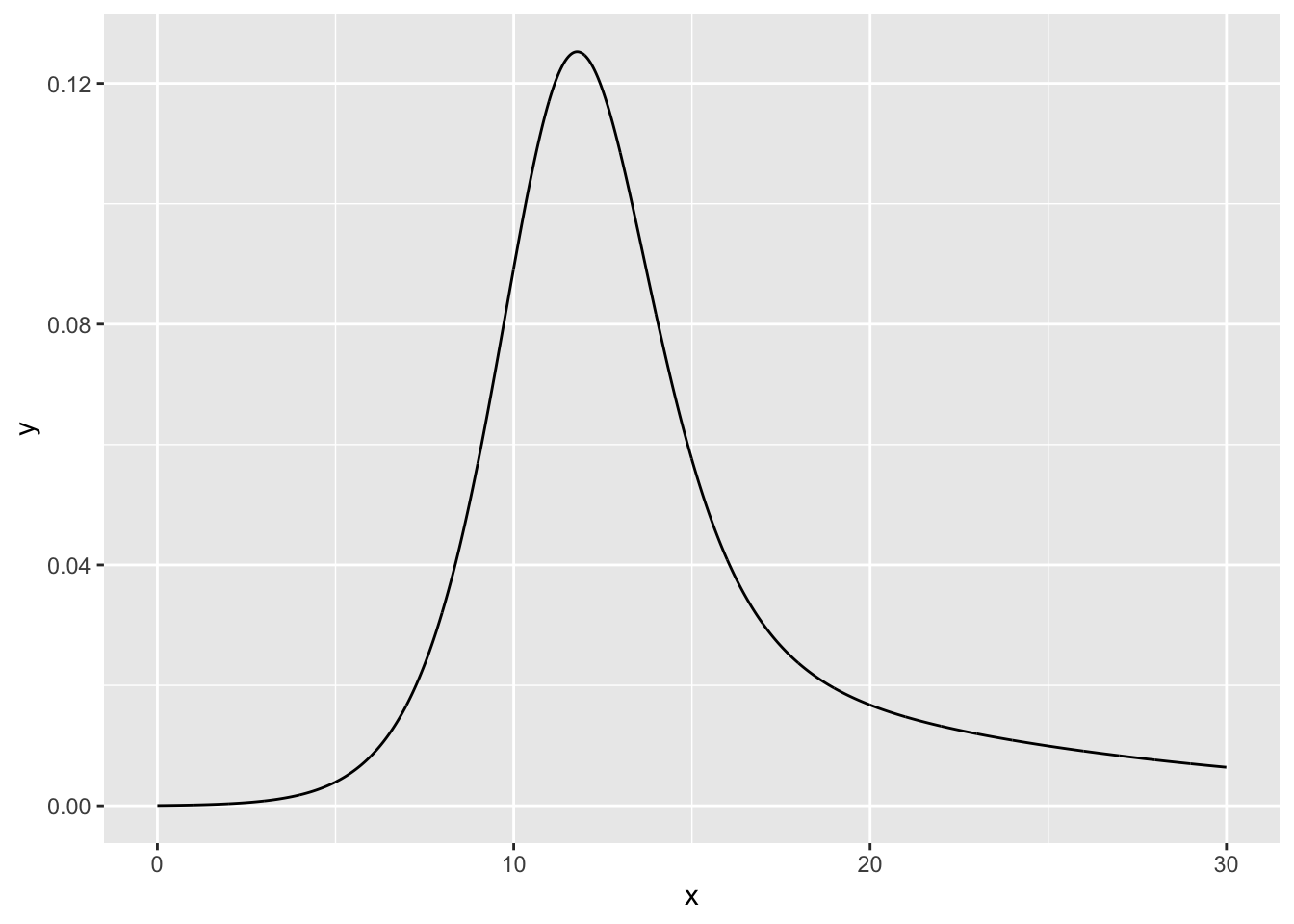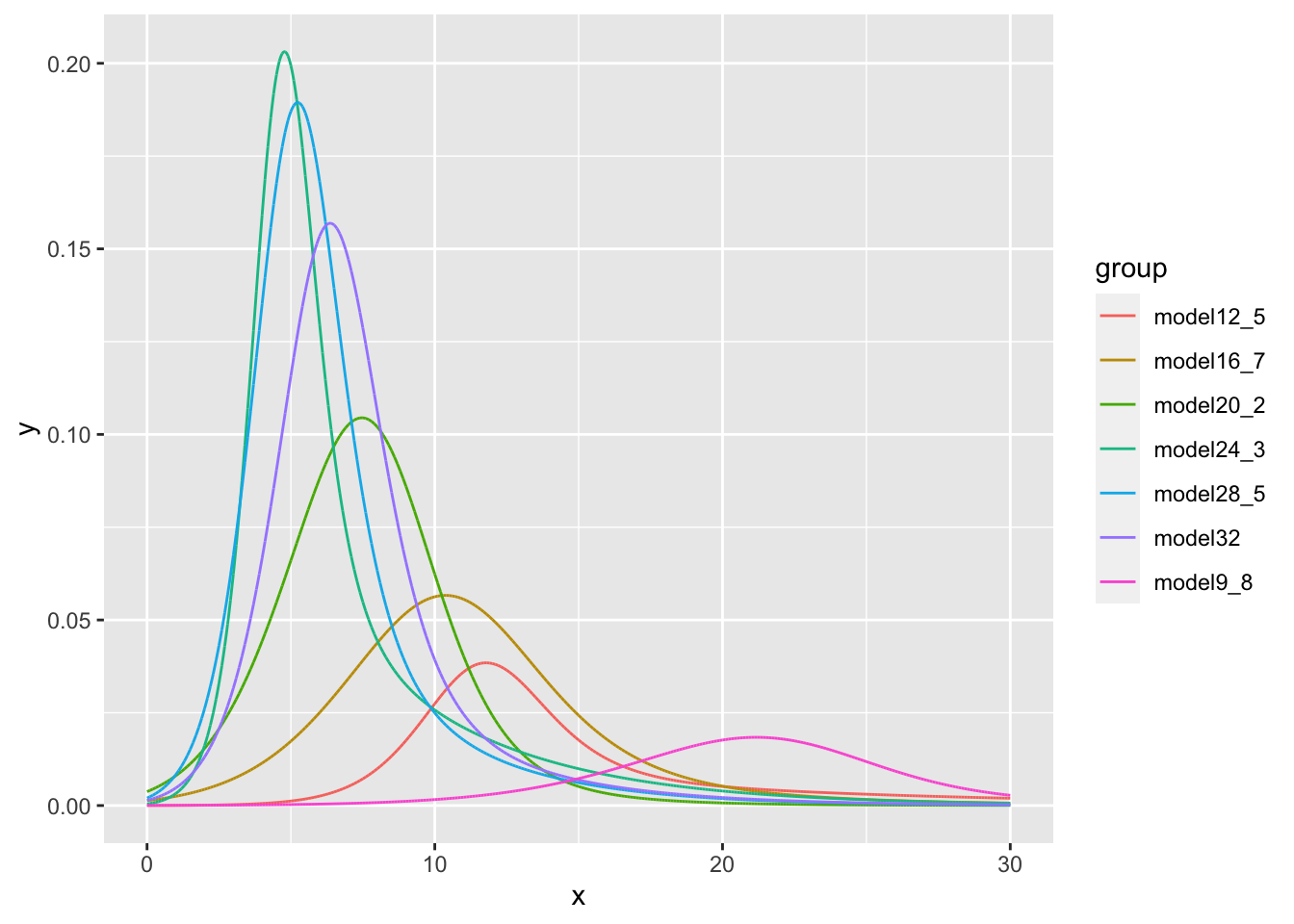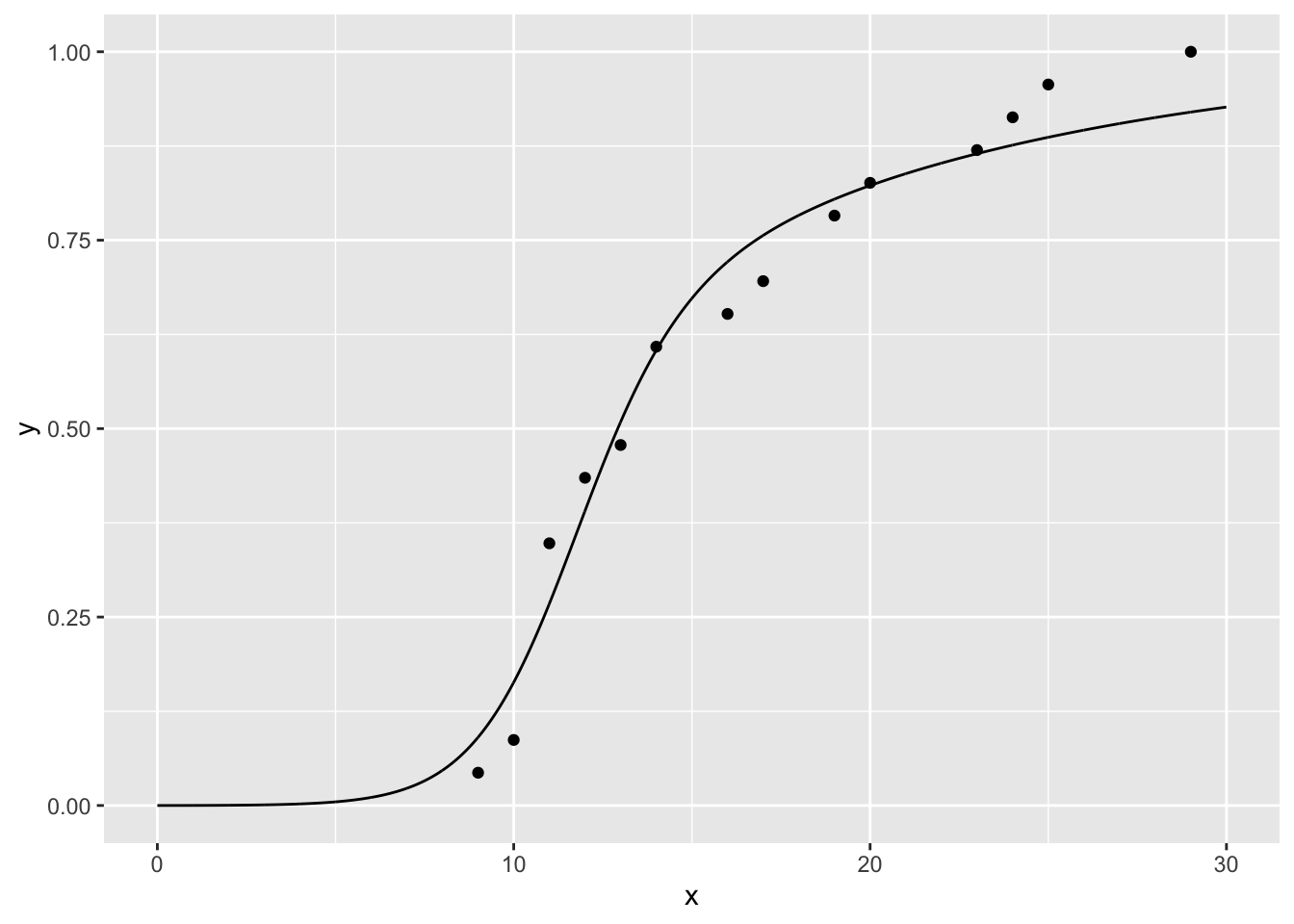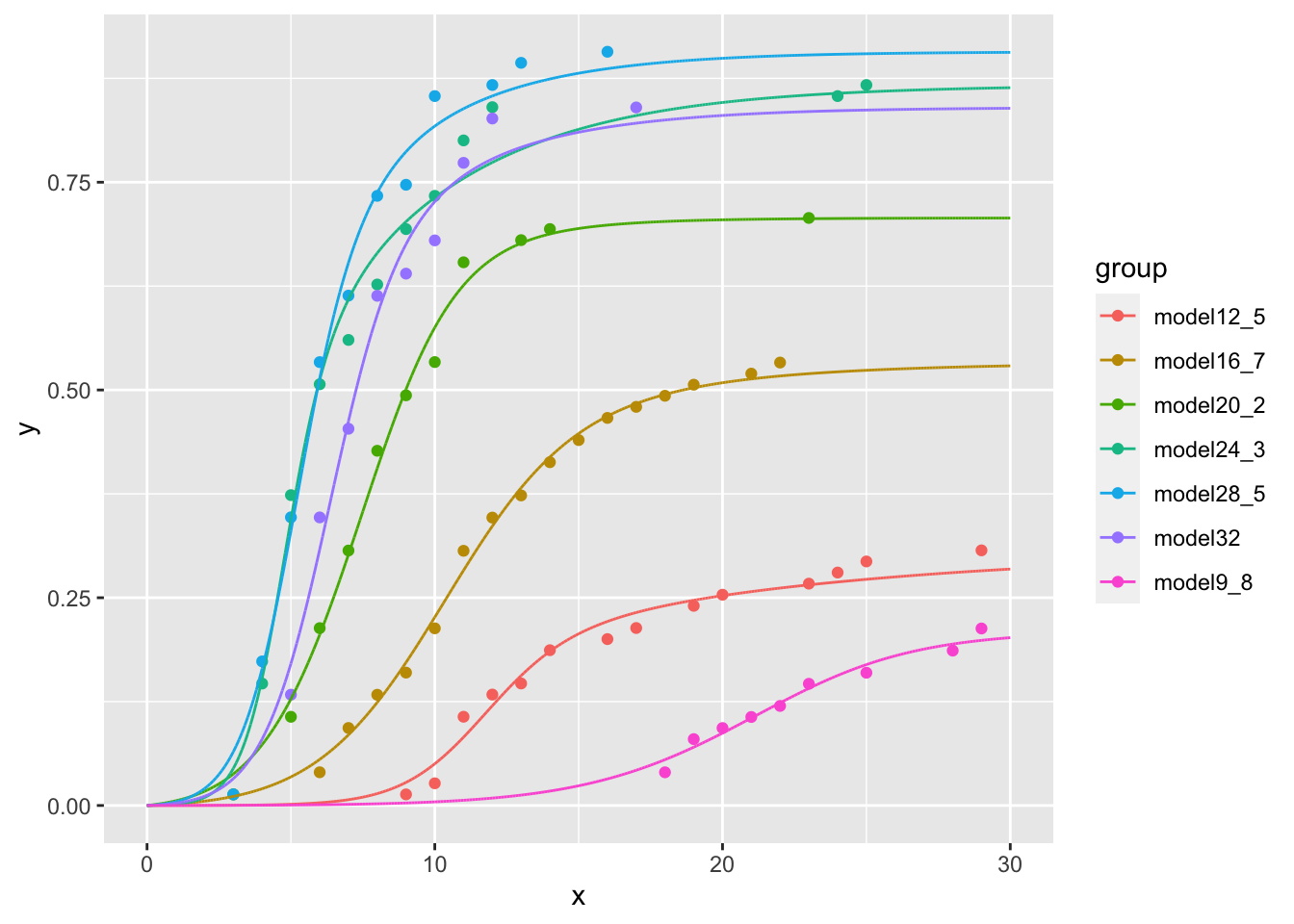# Introduction

This vignette presents the {nlstimedist} package, a method to fit a new distribution model to the time distribution of a biological phenomenon (Franco 2016). The model differentiates between three essential aspects of a time distribution: the rate at which the process is expected to occur (parameter $$r$$), the rate of change of $$r$$ with time, which is reflected in the time concentration of the distribution (parameter $$c$$), and a measure of the overall distribution time lag (parameter $$t$$). The fitting method incorporates the minpack.lm::nlsLM() function (Elzhov et al. 2016) to estimate these three parameters and to plot the estimated time distribution. The {nlstimedist} package, however, also estimates the standard distribution moments. The method is being proposed to analyse the time distribution of biological events such as germination, phenology, invasion, conclusion of a race, etc. Because parameter values have clear, unique effects on three different aspects of the distribution’s shape (and are correlated but not identical to specific moments), they have clear biological interpretation. This allows the user to further investigate the effect that biological (e.g., species, gender, health, etc.) and environmental factors (e.g., temperature) have on a biological time course. For example, are differences between the sexes in the completion of a marathon race reflected in a particular parameter? If so, what do these differences mean in terms of their size, musculature, aerobic capacity, etc.? If the parameters have a biological interpretation, how are they affected by ambient temperature, hydration, sugar levels, etc.?

# Data Setup

In the model, time is represented by variable $$x$$ and the biological phenomenon is represented by variable $$y$$. The values in each $$y$$ column should be proportions and should be calculated from the cumulative number of events. This must be completed for each column in a dataset. If data have been set up in this manner, skip ahead to the modelling section. If the data have not been set up in this format and it is in a raw format of counts vs. time, they must first be cleaned using the tdData() function.

## Cleaning Data Using tdData()

The {nlstimedist} package comes with several example datasets, one being the lobelia dataset.

head(lobelia)
Day Temperature Germination
1   0         9.8           0
2   1         9.8           0
3   2         9.8           0
4   3         9.8           0
5   4         9.8           0
6   5         9.8           0

We can clean and prepare the data for modelling using the tdData() function.

tdLobelia <- tdData(lobelia, x = "Day", y = "Germination", group = "Temperature")
tdLobelia
Day Temperature Germination cumN    propMax
19   18         9.8           3    3 0.18750000
20   19         9.8           3    6 0.37500000
21   20         9.8           1    7 0.43750000
22   21         9.8           1    8 0.50000000
23   22         9.8           1    9 0.56250000
24   23         9.8           2   11 0.68750000
26   25         9.8           1   12 0.75000000
29   28         9.8           2   14 0.87500000
30   29         9.8           2   16 1.00000000
43    9        12.5           1    1 0.04347826
44   10        12.5           1    2 0.08695652
45   11        12.5           6    8 0.34782609
46   12        12.5           2   10 0.43478261
47   13        12.5           1   11 0.47826087
48   14        12.5           3   14 0.60869565
50   16        12.5           1   15 0.65217391
51   17        12.5           1   16 0.69565217
53   19        12.5           2   18 0.78260870
54   20        12.5           1   19 0.82608696
57   23        12.5           1   20 0.86956522
58   24        12.5           1   21 0.91304348
59   25        12.5           1   22 0.95652174
63   29        12.5           1   23 1.00000000
70    3        16.7           1    1 0.02500000
73    6        16.7           2    3 0.07500000
74    7        16.7           4    7 0.17500000
75    8        16.7           3   10 0.25000000
76    9        16.7           2   12 0.30000000
77   10        16.7           4   16 0.40000000
78   11        16.7           7   23 0.57500000
79   12        16.7           3   26 0.65000000
80   13        16.7           2   28 0.70000000
81   14        16.7           3   31 0.77500000
82   15        16.7           2   33 0.82500000
83   16        16.7           2   35 0.87500000
84   17        16.7           1   36 0.90000000
85   18        16.7           1   37 0.92500000
86   19        16.7           1   38 0.95000000
88   21        16.7           1   39 0.97500000
89   22        16.7           1   40 1.00000000
105   5        20.2           8    8 0.15094340
106   6        20.2           8   16 0.30188679
107   7        20.2           7   23 0.43396226
108   8        20.2           9   32 0.60377358
109   9        20.2           5   37 0.69811321
110  10        20.2           3   40 0.75471698
111  11        20.2           9   49 0.92452830
113  13        20.2           2   51 0.96226415
114  14        20.2           1   52 0.98113208
123  23        20.2           1   53 1.00000000
136   3        24.3           1    1 0.01538462
137   4        24.3          10   11 0.16923077
138   5        24.3          17   28 0.43076923
139   6        24.3          10   38 0.58461538
140   7        24.3           4   42 0.64615385
141   8        24.3           5   47 0.72307692
142   9        24.3           5   52 0.80000000
143  10        24.3           3   55 0.84615385
144  11        24.3           5   60 0.92307692
145  12        24.3           3   63 0.96923077
157  24        24.3           1   64 0.98461538
158  25        24.3           1   65 1.00000000
169   3        28.5           1    1 0.01470588
170   4        28.5          12   13 0.19117647
171   5        28.5          13   26 0.38235294
172   6        28.5          14   40 0.58823529
173   7        28.5           6   46 0.67647059
174   8        28.5           9   55 0.80882353
175   9        28.5           1   56 0.82352941
176  10        28.5           8   64 0.94117647
178  12        28.5           1   65 0.95588235
179  13        28.5           2   67 0.98529412
182  16        28.5           1   68 1.00000000
204   5        32.0          10   10 0.15873016
205   6        32.0          16   26 0.41269841
206   7        32.0           8   34 0.53968254
207   8        32.0          12   46 0.73015873
208   9        32.0           2   48 0.76190476
209  10        32.0           3   51 0.80952381
210  11        32.0           7   58 0.92063492
211  12        32.0           4   62 0.98412698
216  17        32.0           1   63 1.00000000

# Modelling the Data

The model is fitted by nonlinear regression employing the Levenberg-Marquardt algorithm. This requires three starting values for $$r$$, $$c$$ and $$t$$, respectively.

## Starting Values

Suggestions for appropriate starting values for each parameter are as follows:

Parameter Recommendation
$$r$$ $$\frac{1}{\text{the period of the time course}}$$, e.g., if completion of the process (all individual events) occurred in 25 days, an appropriate starting value for $$r$$ would be around $$\frac{1}{25}$$ = 0.04.
$$c$$ This requires some trial and error with your particular dataset. We suggest you start with 0.5 and increase (or decrease) it along a logarithmic scale to get a feel of how it is changing. Increasing values of $$c$$ reduce the spread of the distribution: $$c$$ is a measure of concentration of the distribution.
$$t$$ This tends to be close to the mid-point of the monitoring period, but it varies with the skew produced by the combination of parameter values. Nonetheless, as a rule of thumb choose a number near the middle of your time range – if completion of a process (e.g., a marathon race) was closed after 10 hours, choose $$t = 5$$.

## The timedist() Function

The model is fitted to the data using the timedist() function.

# Fitting the model to data already in the format x = time and y = proportion
# of cumulative number of events.
lobelia12_5 <- tdLobelia[tdLobelia$Temperature == 12.5, ] model12_5 <- timedist( lobelia12_5, x = "Day", y = "propMax", r = 0.03, c = 0.5, t = 14.5 ) model12_5 Nonlinear regression model model: propMax ~ 1 - (1 - (r/(1 + exp(-c * (Day - t)))))^Day data: data r c t 0.08339 0.62678 12.09364 residual sum-of-squares: 0.03901 Number of iterations to convergence: 16 Achieved convergence tolerance: 1.49e-08 ## Fixing Starting Values On rare occasions the model may fail to converge within 50 iterations. This may occur if a very small dataset is used. It is possible to overcome this issue by fixing or setting upper and lower bounds for one of the starting values. The parameter $$r$$ is the most appropriate parameter to do this with. It is suggested that you calculate the starting value for $$r$$ as in the starting values section and set the upper and lower bounds around this figure (see below). modelFix <- timedist( data = lobelia12_5, x = "Day", y = "propMax", r = 0.03, c = 0.5, t = 14.5, upper = c(0.1, Inf, Inf), lower = c(0.01, -Inf, -Inf) ) modelFix Nonlinear regression model model: propMax ~ 1 - (1 - (r/(1 + exp(-c * (Day - t)))))^Day data: data r c t 0.08339 0.62678 12.09364 residual sum-of-squares: 0.03901 Number of iterations to convergence: 16 Achieved convergence tolerance: 1.49e-08 # Interpreting the Fit of the Model To assess how well the model has fit the data, and the reliability of parameter estimates, it is suggested that the standard errors, correlations of the estimates, and confidence intervals are obtained. In each example we have used the model model12_5. ## Standard Errors summary(model12_5, correlation = TRUE, symbolic.cor = FALSE) Formula: propMax ~ 1 - (1 - (r/(1 + exp(-c * (Day - t)))))^Day Parameters: Estimate Std. Error t value Pr(>|t|) r 0.083393 0.006615 12.607 6.99e-08 *** c 0.626782 0.156122 4.015 0.00203 ** t 12.093637 0.462246 26.163 2.95e-11 *** --- Signif. codes: 0 '***' 0.001 '**' 0.01 '*' 0.05 '.' 0.1 ' ' 1 Residual standard error: 0.05955 on 11 degrees of freedom Correlation of Parameter Estimates: r c c -0.49 t 0.72 -0.52 Number of iterations to convergence: 16 Achieved convergence tolerance: 1.49e-08 ## Correlation of Parameter Estimates If a higher level of precision is required, the correlation of parameter estimates can be obtained separately. cpe <- vcov(model12_5) cov2cor(cpe) r c t r 1.0000000 -0.4857904 0.7153296 c -0.4857904 1.0000000 -0.5247587 t 0.7153296 -0.5247587 1.0000000 ## Confidence Intervals To produce accurate confidence intervals for the parameters in a nonlinear regression model fit, we can use the confint2() function. confint2(model12_5) 2.5 % 97.5 % r 0.06883386 0.09795187 c 0.28316063 0.97040290 t 11.07624152 13.11103281 ## R-squared There is no direct R-squared for non-linear regression. However, an R-squared value calculated as $$1-\bigg(\frac{\text{Residual Sum of Squares}}{\text{Corrected Sum of Squares}}\bigg)$$ defines a similar quantity for nonlinear regression, is able to describe the proportion of variance explained by the model, and provides a very good estimate of how well the model fits the data. We can extract this value from our model using the tdRSS() function. tdRSS(model12_5)  0.9681957 ## Statistical Moments The following statistical moments for the fitted distribution can be calculated: mean, variance, standard deviation, skew, kurtosis and entropy. model12_5$m$getMoments() mean variance sd skew kurtosis entropy 1 15.75401 83.02729 9.111931 2.897078 12.17524 4.491001 ## Percentiles The percentiles of the distribution can also be calculated. This can be achieved for a single percentile or for a sequence of percentiles. # Extracting a single percentile tdPercentiles(model12_5, n = 0.01) 1% 5.913667 # Extracting a sequence of percentiles from 10% to 90% in steps of 10. tdPercentiles(model12_5, n = seq(0.1, 0.9, 0.1)) 10% 20% 30% 40% 50% 60% 70% 80% 9.159305 10.382816 11.269057 12.073122 12.918504 13.952189 15.516796 18.776037 90% 26.446720  # Plotting the Distribution The package has two built-in graphing functions for plotting the estimated distribution as both a probability density function and a cumulative distribution function. ## Probability Density Function (PDF) The PDF is produced using the function tdPdfPlot(). This function takes one or more objects produced by the model, a scaling parameter S and values for the x-axis xVals (which includes a value for smoothing the curve), as arguments to produce the PDF plot. tdPdfPlot(model12_5, S = 1, xVals = seq(0, 30, 0.01))Multiple models can be plotted on the same graph by providing the function with multiple model objects. # Extract the individual data lobelia9_8 <- tdLobelia[tdLobelia$Temperature == 9.8, ]
lobelia16_7 <- tdLobelia[tdLobelia$Temperature == 16.7, ] lobelia20_2 <- tdLobelia[tdLobelia$Temperature == 20.2, ]
lobelia24_3 <- tdLobelia[tdLobelia$Temperature == 24.3, ] lobelia28_5 <- tdLobelia[tdLobelia$Temperature == 28.5, ]
lobelia32 <- tdLobelia[tdLobelia\$Temperature == 32, ]

# Create the models
model9_8 <- timedist(lobelia9_8, x = "Day", y = "propMax", r = 0.1, c = 0.5, t = 25)
model16_7 <- timedist(lobelia16_7, x = "Day", y = "propMax", r = 0.1, c = 0.5, t = 10)
model20_2 <- timedist(lobelia20_2, x = "Day", y = "propMax", r = 0.1, c = 0.5, t = 10)
model24_3 <- timedist(lobelia24_3, x = "Day", y = "propMax", r = 0.1, c = 1, t = 5)
model28_5 <- timedist(lobelia28_5, x = "Day", y = "propMax", r = 0.1, c = 1, t = 5)
model32 <- timedist(lobelia32, x = "Day", y = "propMax", r = 0.1, c = 0.5, t = 5)

# Generate the plot
tdPdfPlot(
model9_8, model12_5, model16_7, model20_2, model24_3, model28_5, model32,
S = c(0.213, 0.307, 0.533, 0.707, 0.867, 0.907, 0.840),
xVals = seq(0, 30, 0.001)
)## Cumulative Distribution Function (CDF)

The CDF is produced using the function tdCdfPlot(). This function takes one or more objects produced by the model, a scaling parameter S and values for the x-axis xVals (which includes a value for smoothing the curve), as arguments to produce the CDF plot.

tdCdfPlot(model12_5, S = 1, xVals = seq(0, 30, 0.01))tdCdfPlot(
model9_8, model12_5, model16_7, model20_2, model24_3, model28_5, model32,
S = c(0.213, 0.307, 0.533, 0.707, 0.867, 0.907, 0.840),
xVals = seq(0, 30, 0.001)
)